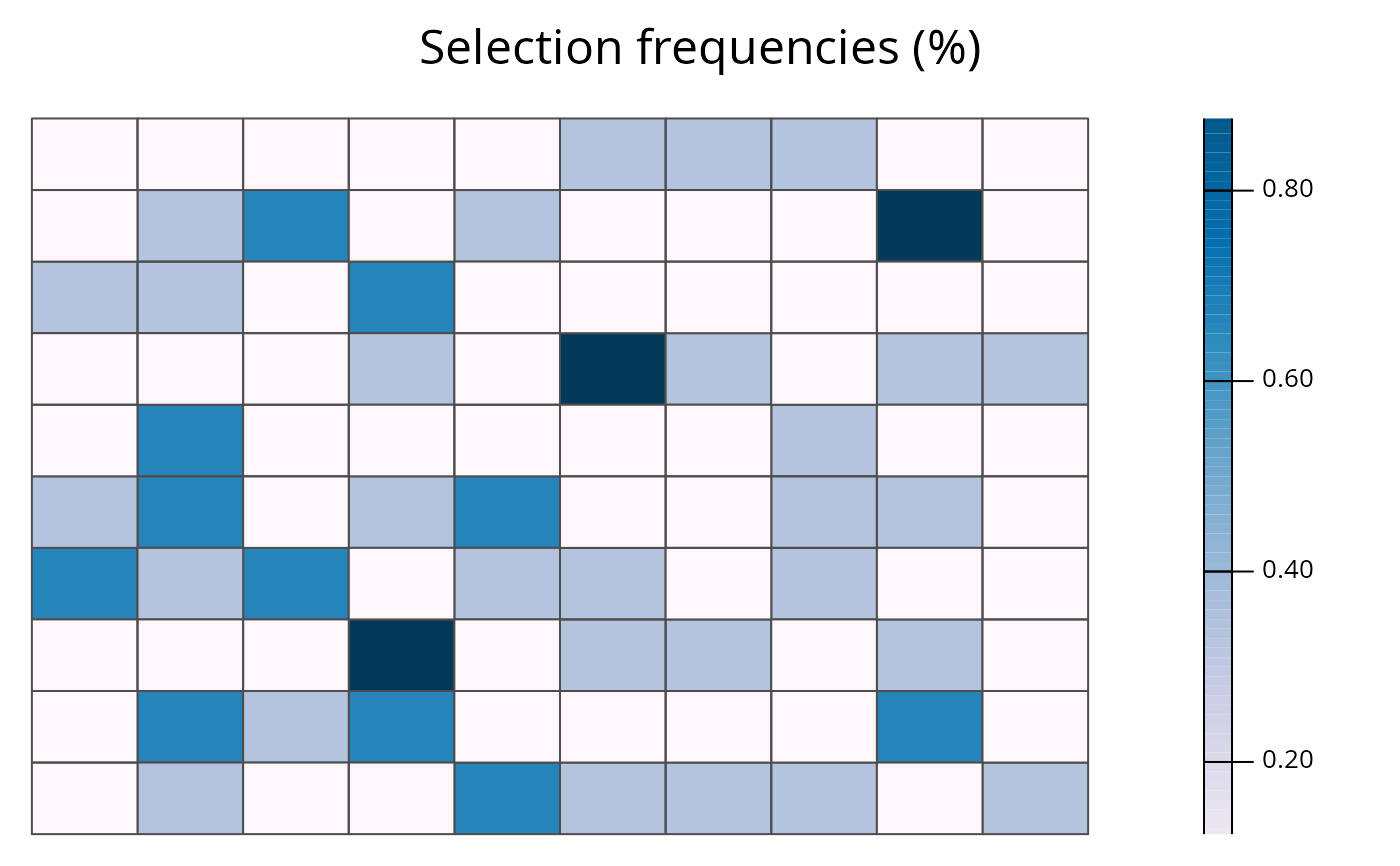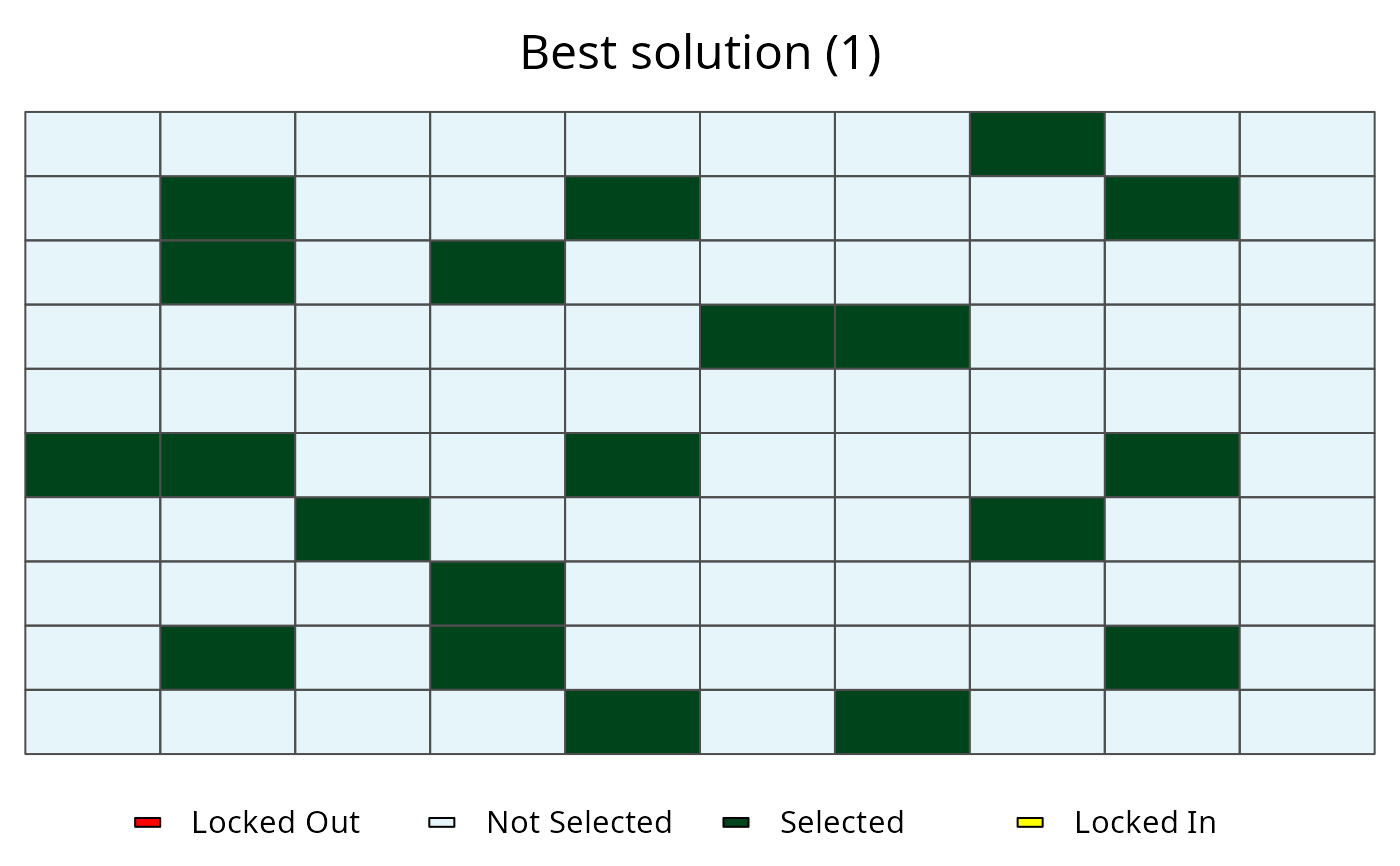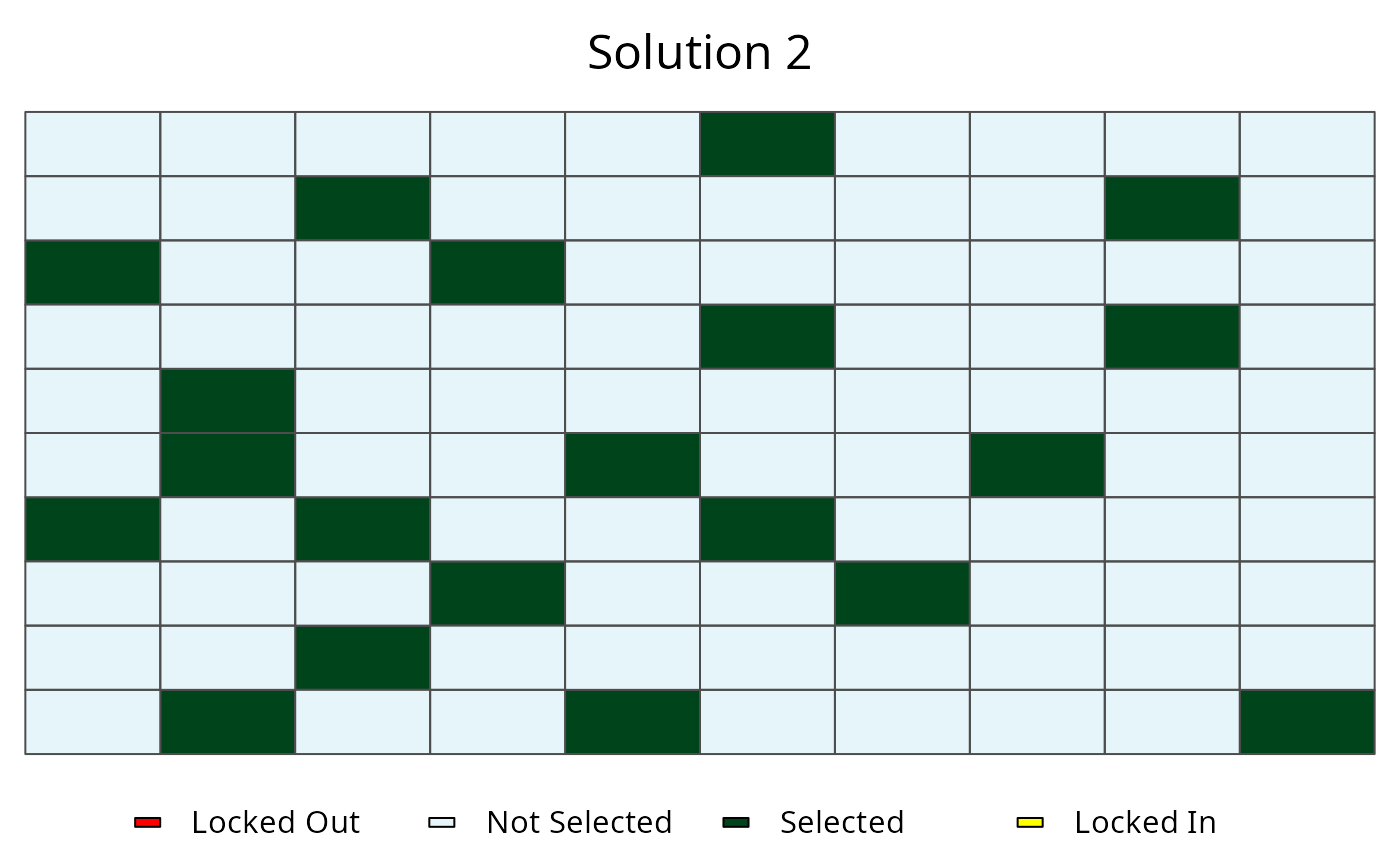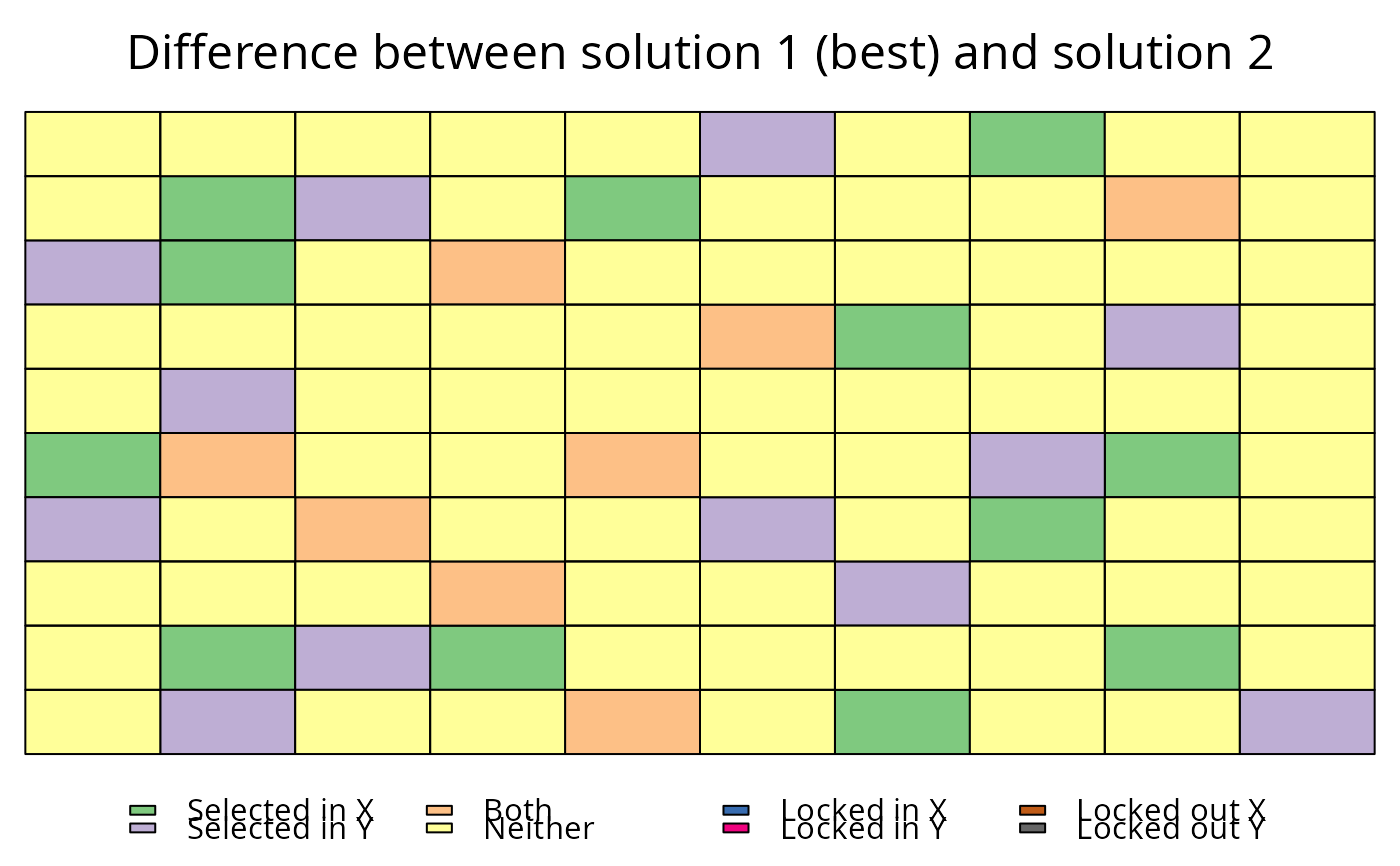This function plots the solutions contained in RapSolved() objects. It can be used to show a single solution, or the the selection frequencies of planning units contained in a single RapSolved() object. Additionally, two RapSolved() objects can be supplied to plot the differences between them.

# S4 method for RapSolved,numeric
plot(x, y, basemap = "none",
pu.color.palette = c("#e5f5f9", "#00441b", "#FFFF00", "#FF0000"), alpha =
ifelse(basemap == "none", 1, 0.7), grayscale = FALSE, main = NULL,
force.reset = FALSE)

# S4 method for RapSolved,missing
plot(x, y, basemap = "none",
pu.color.palette = c("PuBu", "#FFFF00", "#FF0000"),
alpha = ifelse(basemap == "none", 1, 0.7),
grayscale = FALSE, main = NULL,
force.reset = FALSE)

# S4 method for RapSolved,RapSolved
plot(x, y, i = NULL, j = i,
basemap = "none",
pu.color.palette = ifelse(is.null(i), c("RdYlBu", "#FFFF00",
"#FF0000"), "Accent"),
alpha = ifelse(basemap == "none", 1, 0.7),
grayscale = FALSE, main = NULL, force.reset = FALSE)

## Arguments

x

RapSolved() object.

y

Available inputs are: NULL to plot selection frequencies, numeric number to plot a specific solution, 0 to plot the best solution, and a RapSolved() object to plot differences in solutions between objects. Defaults to NULL.

basemap

character object indicating the type of basemap to use (see basemap()). Valid options include "none", "roadmap", "mobile", "satellite", "terrain", "hybrid", "mapmaker-roadmap", "mapmaker-hybrid". Defaults to "none" such that no basemap is shown.

pu.color.palette

character vector of colors to indicate planning unit statuses. If plotting selection frequencies (i.e., j = NULL), then defaults to a c("PuBu", "#FFFF00", "#FF0000"). Here, the first element corresponds to a color palette (per RColorBrewer::brewer.pal()) and the last two elements indicate the colors for locked in and locked out planning units. Otherwise, the parameter defaults to a character vector of c("grey30", "green", "yellow", "black", "gray80", "red", "orange").

alpha

numeric value to indicating the transparency level for coloring the planning units.

grayscale

logical should the basemap be gray-scaled?

main

character title for the plot. Defaults to NULL and a default title is used.

force.reset

logical if basemap data has been cached, should it be re-downloaded?

i

Available inputs are: NULL to plot selection frequencies. numeric to plot a specific solution, 0 to plot the best solution. This argument is only used when y is a RapSolved() object. Defaults to NULL.

j

Available inputs are: NULL to plot selection frequencies. numeric to plot a specific solution, 0 to plot the best solution. This argument is only used when y is a RapSolved() object. Defaults to argument j.

## Details

This function requires the RgoogleMaps package to be installed in order to create display a basemap.

RapSolved().

## Examples

# \dontrun{
# load example data set with solutions
data(sim_rs)

# plot selection frequencies
plot(sim_rs)# plot best solution
plot(sim_rs, 0)# plot second solution
plot(sim_rs, 2)# plot different between best and second solutions
plot(sim_rs, sim_rs, 0 ,2)# }